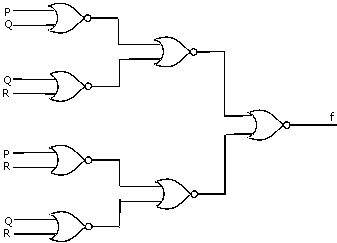Related Articles

# GATE | GATE CS 2010 | Question 65

• Last Updated : 19 Nov, 2018

What is the Boolean expression for the output f of the combinational logic circuit of NOR gates given below?(A) (Q+R)’
(B) (P+Q)’
(C) (P+R)
(D) (P+Q+R)’

The above question contains the NOR gates. Let’s see what NOR gate does.
If A and B are the two inputs to the NOR gate, the NOR gate gives (A+B)’ as the output.

Let’s assign numbers to the Gates for the easy understanding.

```In the 1st column there are 4 NOR Gates,
number them as 1 to 4 ( top to down).

In the 2nd column there are 2 NOR Gates,
number them as 5 and 6 ( top to down).

In the 3rd column there is only 1 NOR Gate,
number it as 7.

1st numbered Gate gives output as : ( P + Q )'
2nd numbered Gate gives output as : ( Q + R )'
3rd numbered Gate gives output as : ( P + R )'
4th numbered Gate gives output as : ( R + Q )'
5th numbered Gate gives output as :
(( P + Q )' + ( Q + R )')'
= ((P + Q)'' . ( Q + R )'') ( De Morgan's law)
= (P + Q ) . ( Q + R ) ( Idempotent Law, A'' = A)
= (PQ + PR + Q + QR )
= (Q(1 + P + R) + PR) = Q + PR ( as, 1 + " any
boolean expression" = 1 )

Similarly 6th numbered Gate gives output as : R + PQ
(as this time R is common here)

Now 7th numbered Gate gives output as :
((Q + PR) + (R + PQ))'
= (Q( 1+P) + R(1+P))'
= (Q+R)' ```

Attention reader! Don’t stop learning now.  Practice GATE exam well before the actual exam with the subject-wise and overall quizzes available in GATE Test Series Course.

Learn all GATE CS concepts with Free Live Classes on our youtube channel.

My Personal Notes arrow_drop_up